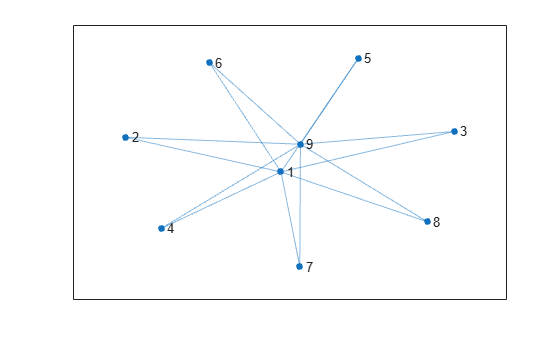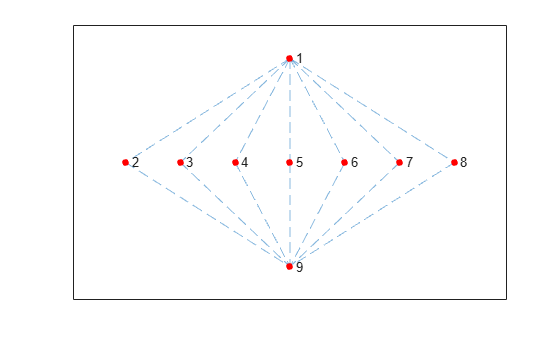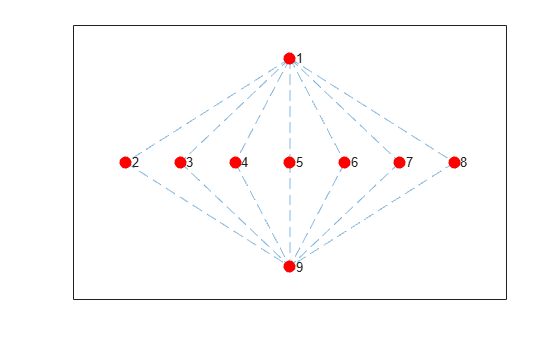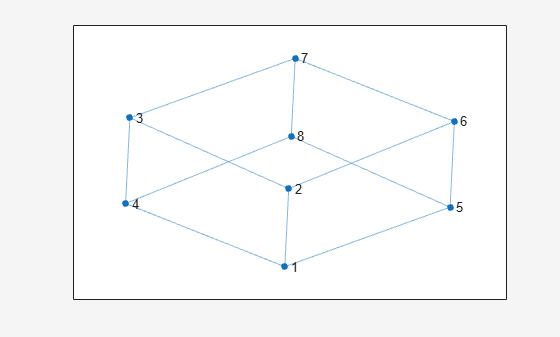# GraphPlot

Graph plot for directed and undirected graphs

## Description

Graph plots are the primary way to visualize graphs and networks created using the `graph` and `digraph` functions. After you create a `GraphPlot` object, you can modify aspects of the plot by changing its property values. This is particularly useful for modifying the display of the graph nodes or edges.

## Creation

To create a `GraphPlot` object, specify an output argument with the `plot` function. For example:

```G = graph([1 1 1 1 5 5 5 5],[2 3 4 5 6 7 8 9]); h = plot(G)```

## Properties

 GraphPlot Properties Graph plot appearance and behavior

## Object Functions

 `layout` Change layout of graph plot `highlight` Highlight nodes and edges in plotted graph `labelnode` Label graph nodes `labeledge` Label graph edges

## Examples

collapse all

Create a `GraphPlot` object, and then show how to adjust the properties of the object to affect the output display.

Create and plot a graph.

```s = [1 1 1 1 1 1 1 9 9 9 9 9 9 9]; t = [2 3 4 5 6 7 8 2 3 4 5 6 7 8]; G = graph(s,t); h = plot(G)``````h = GraphPlot with properties: NodeColor: [0 0.4470 0.7410] MarkerSize: 4 Marker: 'o' EdgeColor: [0 0.4470 0.7410] LineWidth: 0.5000 LineStyle: '-' NodeLabel: {'1' '2' '3' '4' '5' '6' '7' '8' '9'} EdgeLabel: {} XData: [1x9 double] YData: [1x9 double] ZData: [0 0 0 0 0 0 0 0 0] Show all properties ```

Use custom node coordinates for the graph nodes.

```h.XData = [0 -3 -2 -1 0 1 2 3 0]; h.YData = [2 0 0 0 0 0 0 0 -2];```Make the graph nodes red.

`h.NodeColor = 'r';`Use dashed lines for the graph edges.

`h.LineStyle = '--';`Increase the size of the nodes.

`h.MarkerSize = 8;`Use the `savefig` function to save a graph plot figure.

```s = [1 1 1 2 2 3 3 4 5 5 6 7]; t = [2 4 5 3 6 4 7 8 6 8 7 8]; G = graph(s,t); h = plot(G); savefig('cubegraph.fig');``````clear all close all```

Use `openfig` to load the graph plot figure back into MATLAB. `openfig` also returns a handle to the figure, `y`.

`y = openfig('cubegraph.fig');`

Use the `findobj` function to locate the correct object handle using one of the property values. Using `findobj` allows you to continue manipulating the original `GraphPlot` object used to generate the figure.

`h = findobj('Marker','o')`
```h = GraphPlot with properties: NodeColor: [0 0.4470 0.7410] MarkerSize: 4 Marker: 'o' EdgeColor: [0 0.4470 0.7410] LineWidth: 0.5000 LineStyle: '-' NodeLabel: {'1' '2' '3' '4' '5' '6' '7' '8'} EdgeLabel: {} XData: [-1.2777 0.3428 2.0062 0.5490 -2.0093 -0.5481 1.2810 -0.3440] YData: [1.2916 -0.3562 0.5243 2.0057 -0.5235 -2.0034 -1.2921 0.3537] ZData: [0 0 0 0 0 0 0 0] Show all properties ```

## Compatibility Considerations

expand all

Behavior changed in R2018b

Behavior changed in R2018a